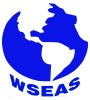Conference TopicsContributions related, but not limited, to the following topics of interest are welcome:

Linear Algebra and Applications:
Matrix Theory,Tensor Analysis, Multilinear Algebra, Spaces over fields or rings, Tensor algebras or subalgebras, Nonnegative matrices, Inequalities in linear algebra, Combinatorial linear algebra, Matrix numerical linear analysis, Representation theory, Lie theory, Invariant theory, Functional analysis, Computational Linear Algebra, Markov Chains, Iterative methods, Error Estimation in Iterative Methods, Eigenvalue Problems, Componentwise and Structured Perturbations, Convex Optimization, Approximation of Large-scale Dynamical Systems, Large Scale Systems, Identification, Linear Algebra in Simulation and Mathematical Biology, Linear Algebra Information Retrieval and Management, Totally Nonnegative Matrices, Special Topics and Applications.

Numerical Analysis and Applications:
Numerical Methods for Solving Equations, Polynomial Factorization, Numerical Methods for Ordinary and Partial Differential Equations, Numerical Methods for Integral Equations, Numerical Methods for Integral-Differential Equations, Numerical Methods for Algebro-Differential Equations, Numerical Methods for Singular Equations, Numerical Linear Algebra, Numerical Behaviour of Optimization Algorithms, The Art of Computer Programming of Numerical Methods, Parallel Computing, Distributed Computing, Supercomputing, Finite Elements, Mathematical Aspects of Scientific Computing, Error Analysis, Stability Problems, Convergence Problems, High Complexity Numerical Methods, Numerical Mathematics for Non-linear Systems Study, Numerical Computation in Dynamical Systems and Chaos, Simulation, Numerical Analysis problems in Science and Engineering, Special Topics and Applications.

Differential Equations and Applications:
Ordinary differential equations, Partial differential equations, Stochastic differential equations, Topological dynamics, Integral equations, functional differential equations, Differential Equations in Sobolev Spaces, Variational methods in ODEs and in PDEs, Existence, Multiplicity results for solutions to ODEs and PDEs, Nonlinear Systems, Chaos, Morse theory, Manifolds, Bifurcation and Degree theory, Singular perturbation problems, Qualitative theory (stability, periodicity, boundedness, etc.), Difference Equations, Numerical Methods for ODEs and PDEs, Diff.Equations in Science and Engineering, Special Topics and Applications.

Probabilities, Statistics, Operational Research:
Theoretical Probability Theory, Applied Probability Theory, Stochastic Processes, Mathematical Statistics, Applied Statistics, Game Theory, Prediction Theory, Estimation Theory, Identification, Simulation, Mathematical Programming, Statistical Techniques, Continuous and Discrete optimization, Stochastic models, Optimization theory, Multi-person decisions, New Theories in Operational Research, Queueing Theory, Reliability Theory, Routing Theory, Measurement Theory, Marketing and Production Organization, Transportation Systems, Telecommunication theory, Applications in Computer engineering, Epidemiology, Financial mathematics, Information systems and traffic management, Inventory theory, Scheduling, Management Science, Applications in Science and Engineering,Related Topics

Optimization and Applications:
Optimization Theory, Linear Programming, Quadratic Programming, Convex Programming, Nonlinear Programming, Complementarity problems, Stochastic Programming, Combinatorial Programming, Integer Programming, Convex, Nonsmooth and Variational analysis, Multiobjective programming, Game Theory, Algorithms for parallel architectures, Global Optimization, Neural Networks for Optimization, Evolutionary Computing, Genetic Algorithms, Optimal Control, Stochastic Control, Variational Principles and Applications, Software Development for Optimization, Heuristic Algorithms for Optimization, Tabu Methods, Simulated Annealing, Computational Analysis of Optimization Algorithms

Algorithms, Discrete Mathematics, Systems, Communications, Control:
Algorithms Theory: New Problems - New Theories, Computational Complexity, Convergence, Error Analysis, Data structures, Algorithms on graphs, Arithmetic algorithms, Combinatorial searches and objects, Discrete optimization, Geometric algorithms, Methods of algorithmic analysis, New algorithms and data structures, New analyses or comparisons of known algorithms, Semantics, Predictive Semantics, Randomized Algorithms, Equational logic programming, Functional Languages, Parallel and Distributed Computation, Modern Problems in Discrete mathematics, Combinatorics and Graph Theory, Functional systems theory, Coding, Cryptology, Object-Oriented Programming, Computerised Signal Processing, Computer Graphics, Computational Geometry, Industrial Systems, Real Time Systems, Multimedia, Probability problems of discrete mathematics, Discrete structures, Extremal problems, Posets, Enumeration problems, Network algorithms, Combinatorial Optimization and Mathematical Programming, Network optimization, Integer programming, Approximation algorithms, Randomized algorithms, Theoretical Computer Science, Coding and Information Theory, Error-correcting codes, Data compression, Switching networks, Communication protocols, Number theory, Group Theory and Applications, Ideal Theory and Applications, Ring Theory and Applications, Field Theory and Applications, Galois Theory and Applications, Systems Theory, Control Systems, Robotics, Computational Intelligence, Neural Networks, Fuzzy Logic Systems and technology, Knowledge Modelling, Decision Support Systems, Hierarchical Control Systems, Aerospace Systems, Stochastic Systems, Non-linear Systems, Telecommunication Systems, Information Systems, Signal Processing Systems, Multidimensional Systems, Multivariable systems, Hybrid Systems, Multirate Systems, Speech and Image Processing Systems, Discrete Event Dynamic Systems, Manufacturing Systems, Decentralised Systems, Remote Sensing, Human-Machine Systems, Sonar and underwater acoustic systems, Undersea Systems, Navigation and Tracking Systems, Space Systems, Wavelets, Verification and Validation, Virtual Reality, Computer Vision, Computer Algebra, Symbolic Computation, Adaptive and Learning Systems, Classification, Identification, Mathematical Control Theory, Telecommunications, Communication Theory, Security, Protocols, Microwaves, Antennas, Radar, Propagation, Related Topics

## Bulletin Board

Currently:

The Conference Guide is online.

The paper submission deadline has expired. Please choose a future conference to submit your paper.